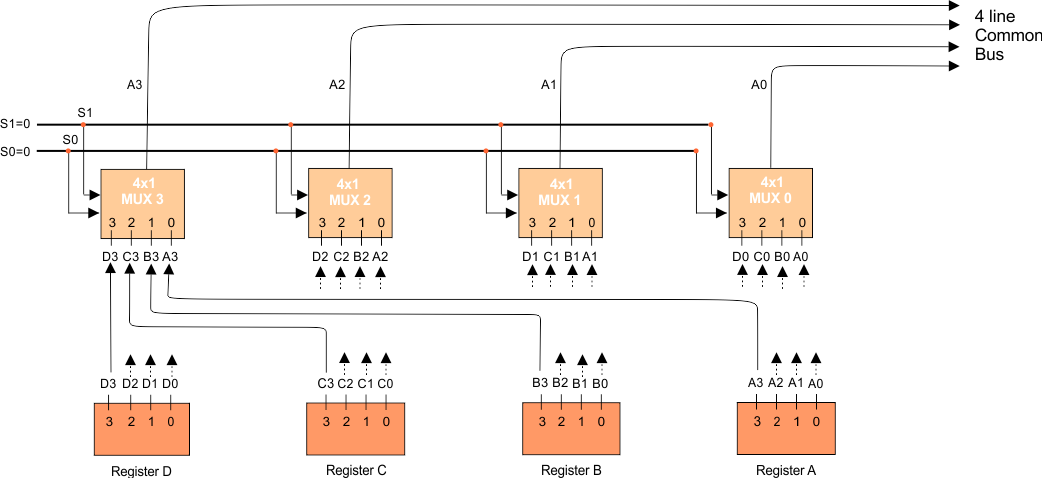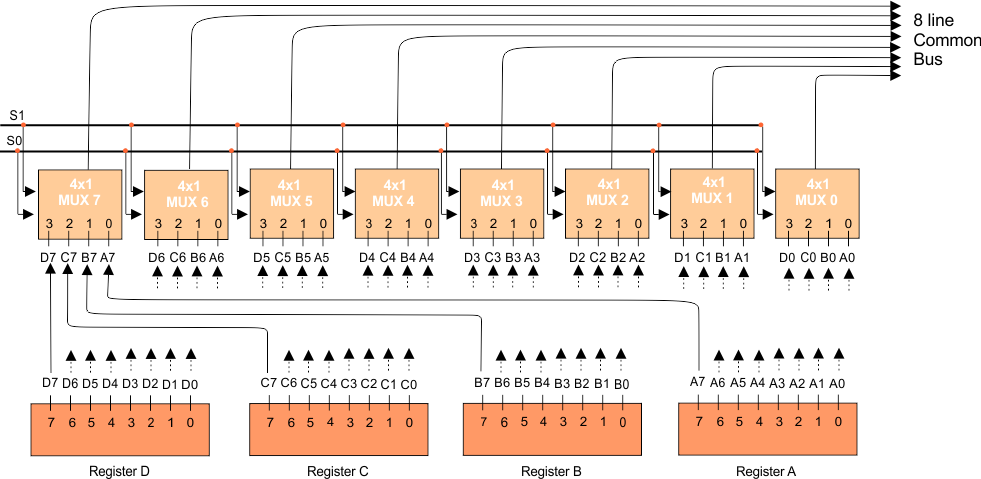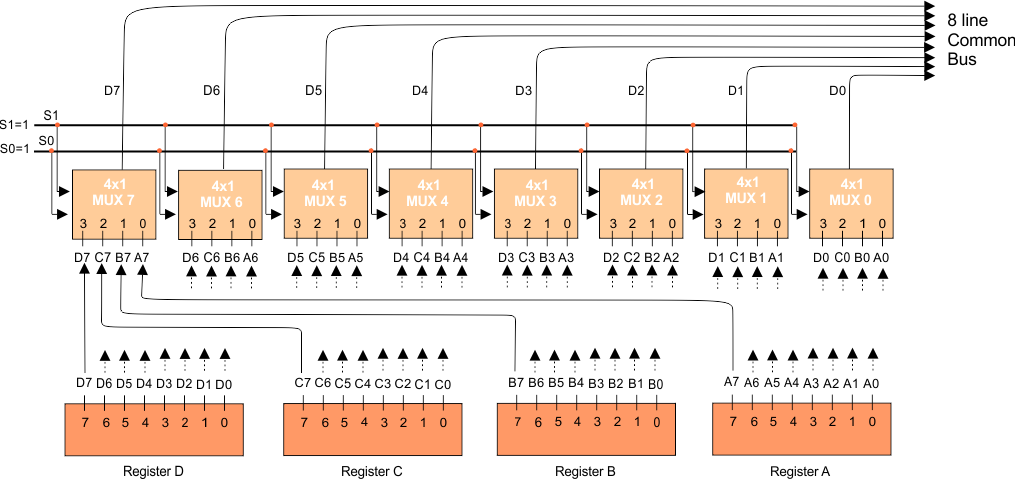Select Page

Computer Organization

Memory Organization
Interrupt
DMA Controller

# Common Bus System (4-bit, 8-bit)

Generally, computers use many registers for different purposes. We need to transfer the data and instruction between these registers. So, To transfers the data between the registers, the common bus system is used . For this, we connect all registers with a common BUS through Multiplexer.

In this article, we will learn about 4-bit and 8-bit registers architecture but mostly registers are 16-bit in computer architecture. The concept of working of common bus system in 4-bits, 8bits or 16 bits is almost same.

## 4-bit Register in the Common Bus System

4-bit register means that size of the register is 4 bit. These 4-bit register uses 4 multiplexers because no. of bits in register is always equal to no. of multiplexer. This mechanism is also known as 4×1 multiplexer. 4×1 Multiplexer means that there are four inputs to each multiplexer form four registers and one output to the common bus.

As in the above diagram, There are 4 registers and 4-Multiplexers, each register is of 4 bits, numbered 0 to 3. There are 2 select lines S0 and S1. These lines are connected to the select inputs of the multiplexers from registers.

## How Registers and multiplexers are connected with each other?

The bits of Register A are (A3, A2, A1, A0). Register B is (B3, B2, B1, and B0).  Register C is (C3, C2, C1, and C0). And Register D is (D3, D2, D1, and D0).

And the first Multiplexer is (D0, C0, B0, and A0). The second Multiplexer is (D1, C1, B1, and A1). The third Multiplexer is (D3, C3, B3, and A3). The fourth Multiplexer is (D4, C4, B4, and A4).

If we connect the first multiplexer with registers then fetch all zero bits from all registers and connect them to the first multiplexer. And in some way, if we want to connect the second multiplexer with registers then fetch all bits from position 1 of all registers and connect them to 2nd multiplexer and so on for other register and multiplexer connection.

## How the bits are transferred from multiplexer to common bus?

We give values to select lines and select lines are connected with every multiplexer. So the value of the select line activate the relevant bit of each multiplexer and transfer it to common bus. A table for all combinations of select lines (S1S0) is given below.

When S1S0=00, then Register A activated. As select lines are connect to every multiplexer. So, it activates the zero (0) bit of every multiplexer because Zero bit of each multiplexer is connect with register A. So one output from each multiplexer will send to common bus as shown in the following diagram.

#Output A3 from Multiplexer 3, A2 from Multiplexer 2, A1 from Multiplexer 1, and A0 from Multiplexer 0, arrives at common bus from 4 multiplexers. Which are the values of register A. So, data of register A is load now in common bus which can access by any other register in the system, or any other part of system i.e. ALU, memory, etc. And so on for other registers.

Look and another example, when S1S0=11 then register D selected, and it can transfer data to the common bus. Because on S1S0=11, 3rd data inputs of all the multiplexers are applied to the common bus. As we know, 11 in a binary number system is equal to 3 in decimal.

As the 3rd data inputs of all the multiplexers receive the inputs from the register D, so register D gets selected. Just like the same case with other registers.

```Number of multiplexers needed = Number of bits in each register
Number of inputs in each multiplexers needed = Total Number of registers.```

## Explanation of 8×1 Multiplexer

If the register size is 8 Bit and there are four registers then a connection to the common bus is shown in the following diagram.

#As there are 4 registers. So, No. of inputs to each multiplexer is 4 and the total no of bits in each register is 8. So, the total multiplexer will be 8. As there are four registers So, two select lines (two bits) are enough to represent these four registers.

## 8×1 Multiplexer with Example

Let suppose, when S1S0= 11 then Register D will activate and 1 output from each multiplexer of Register D to the common bus is shown in the following diagram.

#Question: How registers communicate with each other through a common bus?

Answer: We connect all registers with a common bus through the multiplexers.

Note: If a common bus has 8 registers of 16 bits then it requires 16 multiplexers. Each multiplexer will contain eight data input lines and three selection lines (S2, S1, and S0).

Help Other’s By Sharing…